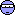Topic: MFinest

Are there any plans to support MFinest in libflowgpu too?

Re: MFinest

I think No.
Finest-clip is not necessary for GPU.
It works without it.

Re: MFinest

Oh ok. I was comparing them and it seems that MFinest adds some nice sharpness to the frame; retains more details. I thought it would be a cool additionRe: MFinest

With pel>1 MSmoothFPS does Finest-like calculations inside GPU.Re: MFinest

GPU vs SSE.
You see the SSE one is sharper with small details, like the trees to the right, and the truck in the lower-middle

Re: MFinest

Can you show text of this script?

Re: MFinest

SSE:

Input = DSS2("filename")
backward = MAnalyse(Super, blksize=16, searchparam=4, plevel=0, isb=true , badSAD=1000, overlap=4, search=3, dct=5, pelsearch=3)
forward  = MAnalyse(Super, blksize=16, searchparam=4, plevel=0, isb=false, badSAD=1000, overlap=4, search=3, dct=5, pelsearch=3)
backward = MRecalculate(Super, backward, blksize=8, searchparam=1, overlap=2, search=3, dct=5)
forward  = MRecalculate(Super, forward,  blksize=8, searchparam=1, overlap=2, search=3, dct=5)
svp_flow_lib = "C:\path\libflowsse.dll"
Finest = MFinest(Input, Super)
MSmoothFps(Input, Super, backward, forward, finest=Finest, num=48000, den=1001, algo=23, ml=0, sadml=150, sadgamma=2, block=false, blend=true)

GPU:

Input = DSS2("filename")
backward = MAnalyse(Super, blksize=16, searchparam=4, plevel=0, isb=true , badSAD=1000, overlap=4, search=3, dct=5, pelsearch=3)
forward  = MAnalyse(Super, blksize=16, searchparam=4, plevel=0, isb=false, badSAD=1000, overlap=4, search=3, dct=5, pelsearch=3)
backward = MRecalculate(Super, backward, blksize=8, searchparam=1, overlap=2, search=3, dct=5)
forward  = MRecalculate(Super, forward,  blksize=8, searchparam=1, overlap=2, search=3, dct=5)
svp_flow_lib = "C:\path\libflowgpu.dll"
MSmoothFps(Input, Super, backward, forward, num=48000, den=1001, algo=23, ml=0, sadml=150, sadgamma=2, block=false, blend=true)

Re: MFinest

Thank you. You are right.
I see that results of these scripts are different.I think there are two problems:
1) Different motion compensation phases
2) Subpixel motionblur on GPU

Re: MFinest

Cool gifIs it something that can be fixed/improved?

Re: MFinest

SubJunk
This question to Chainik.
I think yes. We will try to fix both problems.

Re: MFinest

Yes, I must confirm that the problem exists - there's a small (pixel or half-pixel) difference in interpolated vectors (and masks) between SSE and GPU modes.
But only with overlapped blocks.

SubJunk
If you turn off overlapping, do you see any difference?

Re: MFinest

They are still different with overlapping removed

Re: MFinest

Hmm. May be it depends on the video frame size.
I've tested on 640*360.

Re: MFinest

Well my example isn't as good as MAG's one, but if you want the testing video I use it is here.
It is just a video with some scenes from different movies and TV shows that are difficult for interpolation scripts to handle, so it is useful for tweaking scriptsThe part where the SSE vs GPU difference is most obvious is from 0:44 to 0:52, and that is where the screenshots from here came fromIt looks like in this case MAG's source would be more useful, though, since his example is much more obvious.

Re: MFinest

I think that there's no issue when there're integer number of blocks in width (and height).

640*360 with 8*8 - 80*45 blocks, and it's OK.
But 640*360 with 8*8 and overlap 2*2 - 160.66*60 blocks

And I hope I know whyRe: MFinest

SubJunk
The new 3.0.1 version doesn't resolve the issue. I'm working on it, wait for 3.0.2Re: MFinest

CoolIs there a changelog for 3.0.1?